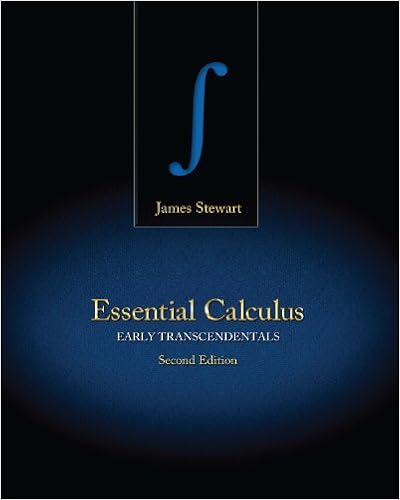# Sequences_and_Series - 11 Sequences and Series Consider the...

• 42

This preview shows page 1 - 4 out of 42 pages.

##### We have textbook solutions for you!
The document you are viewing contains questions related to this textbook.The document you are viewing contains questions related to this textbook.
Chapter 8 / Exercise 14
Essential Calculus: Early Transcendentals
StewartExpert Verified
11 Sequences and Series Consider the following sum: 1 2 + 1 4 + 1 8 + 1 16 + · · · + 1 2 i + · · · The dots at the end indicate that the sum goes on forever. Does this make sense? Can we assign a numerical value to an infinite sum? While at first it may seem di cult or impossible, we have certainly done something similar when we talked about one quantity getting “closer and closer” to a fixed quantity. Here we could ask whether, as we add more and more terms, the sum gets closer and closer to some fixed value. That is, look at 1 2 = 1 2 3 4 = 1 2 + 1 4 7 8 = 1 2 + 1 4 + 1 8 15 16 = 1 2 + 1 4 + 1 8 + 1 16 and so on, and ask whether these values have a limit. It seems pretty clear that they do, namely 1. In fact, as we will see, it’s not hard to show that 1 2 + 1 4 + 1 8 + 1 16 + · · · + 1 2 i = 2 i 1 2 i = 1 1 2 i 255
##### We have textbook solutions for you!
The document you are viewing contains questions related to this textbook.The document you are viewing contains questions related to this textbook.
Chapter 8 / Exercise 14
Essential Calculus: Early Transcendentals
StewartExpert Verified
256 Chapter 11 Sequences and Series and then lim i →∞ 1 1 2 i = 1 0 = 1 . There is one place that you have long accepted this notion of infinite sum without really thinking of it as a sum: 0 . 3333 ¯ 3 = 3 10 + 3 100 + 3 1000 + 3 10000 + · · · = 1 3 , for example, or 3 . 14159 . . . = 3 + 1 10 + 4 100 + 1 1000 + 5 10000 + 9 100000 + · · · = π . Our first task, then, to investigate infinite sums, called series , is to investigate limits of sequences of numbers. That is, we o cially call i =1 1 2 i = 1 2 + 1 4 + 1 8 + 1 16 + · · · + 1 2 i + · · · a series, while 1 2 , 3 4 , 7 8 , 15 16 , . . ., 2 i 1 2 i , . . . is a sequence, and i =1 1 2 i = lim i →∞ 2 i 1 2 i , that is, the value of a series is the limit of a particular sequence. While the idea of a sequence of numbers, a 1 , a 2 , a 3 , . . . is straightforward, it is useful to think of a sequence as a function. We have up until now dealt with functions whose domains are the real numbers, or a subset of the real numbers, like f ( x ) = sin x . A sequence is a function with domain the natural numbers N = { 1 , 2 , 3 , . . . } or the non-negative integers, Z 0 = { 0 , 1 , 2 , 3 , . . . } . The range of the function is still allowed to be the real numbers; in symbols, we say that a sequence is a function f : N R . Sequences are written in a few di ff erent ways, all equivalent; these all mean the same thing: a 1 , a 2 , a 3 , . . . { a n } n =1 { f ( n ) } n =1 As with functions on the real numbers, we will most often encounter sequences that can be expressed by a formula. We have already seen the sequence a i = f ( i ) = 1 1 / 2 i ,
11.1 Sequences 257 and others are easy to come by: f ( i ) = i i + 1 f ( n ) = 1 2 n f ( n ) = sin( n π / 6) f ( i ) = ( i 1)( i + 2) 2 i Frequently these formulas will make sense if thought of either as functions with domain R or N , though occasionally one will make sense only for integer values. Faced with a sequence we are interested in the limit lim i →∞ f ( i ) = lim i →∞ a i . We already understand lim x →∞ f ( x ) when x is a real valued variable; now we simply want to restrict the “input” values to be integers. No real di ff erence is required in the definition of limit, except that we specify, per- haps implicitly, that the variable is an integer. Compare this definition to definition 4.10.4.
•••